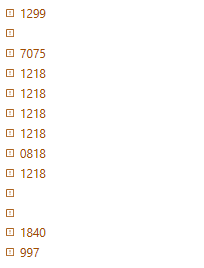# Help me to create regex to only get numbers

You want only 8515 value or anything more @StevenIsRobotOnline

i want to get the numbers only based on those use cases pattern if possibile

Okay Got it @StevenIsRobotOnline

``````=> Assign -> StrOutput = System.Text.RegularExpressions.Regex.Match(StrVar.ToString,“((?<=(^[A-Z]+\s+[A-Z]+)|([A-Z]+\s+[A-Z]+)|[A-Z]+\s+[A-Z]+\s+|[A-Z]+\s+[A-Z]+\-)\d+)”).Value
``````

It’s working as expected check now

@StevenIsRobotOnline

``````System.Text.RegularExpressions.Regex.Match(Inputvariable.ToString,"(((?<=[A-Z]+\s+[A-Z]+\s*.?)\d+))").Value
``````

Regards

Hi Thanks for the hel but it still wont work properly on some of my use cases

1.WILDUN DUMA 8515 → 8515
2.WILWAL SE1865 GEN+ Feed, Food (N570) - >570
3.WILWAL AI1865 GEN+ Feed, Food (N570) ->570
4.WILDUN1840GKP+FEED,FOOD(I570)/RSPOMB ->1840

thank you

@StevenIsRobotOnline

``````System.Text.RegularExpressions.Regex.Match(Inputvariable.ToString,"(((?<=[A-Z]+\s?[A-Z]+\s*.?)\d+))").Value
``````

1 Like

Use the below one

``````=> Assign -> StrOutput = System.Text.RegularExpressions.Regex.Match(StrVar.ToString,“((?<=(^[A-Z]+\s+[A-Z]+)|([A-Z]+\s+[A-Z]+)|[A-Z]+\s+[A-Z]+\s+|[A-Z]+\s+[A-Z]+\-)\d+)|(?<=[A-Z]+)\d+(?=[A-Z])”).Value
``````

Hi , somehow still missing some value with the same use casestruth is the solution from vrdabberu works ,

but if you have a solution which can get same output with same accuracy i will tag yours as solution

@StevenIsRobotOnline

Can you please send me all the required ouputs?

Regards

Is my regex expression is not working for you @StevenIsRobotOnline

Check the below one. Let me know if it’s now working

@StevenIsRobotOnline

Could please provide me all the input and output texts.

Regards

@StevenIsRobotOnline

``````=> Assign -> StrOutput = System.Text.RegularExpressions.Regex.Match(StrVar.ToString,"(((?<=[A-Z]+\s*[A-Z]+\s+.?)\d+)|((?<=[A-Z]+\s+[A-Z]+\d+\s+[A-Z]+.?\s+[A-Za-z]+.?\s+[A-Za-z]+\s+.?[A-Z]+)\d+)|((?<=[A-Z]+\s*[A-Z]+)\d+(?=\s*\$))|((?<=[A-Z]+\s*[A-Z]+)\d+(?=[A-Z]\s*\$))|((?<=[A-Z]+\s+[A-Z]+)\d+(?=[.,|@]))|((?<=[A-Z]+)\d+(?=[A-Z]+))|((?<=[A-Z]+\s+[A-Z]+\-)\d+)|((?<=[A-Z]+[.,@|-]+)\d+)|((?<=[A-Z]+\s+[A-Z]+)\d+(?=\s+[A-Z]+\d+))((?<=[A-Z]+\s+[A-Z]+)\d+(?=\s*\$)))").Value
``````

Hope it helps!!

finnally , thank you so much

1 Like

This topic was automatically closed 3 days after the last reply. New replies are no longer allowed.